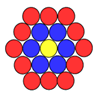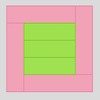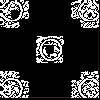#### You may also like### Counting Counters

Take a counter and surround it by a ring of other counters that MUST touch two others. How many are needed?### Cuisenaire Squares

These squares have been made from Cuisenaire rods. Can you describe the pattern? What would the next square look like?### Doplication

We can arrange dots in a similar way to the 5 on a dice and they usually sit quite well into a rectangular shape. How many altogether in this 3 by 5? What happens for other sizes?

# Crossings

##### Age 7 to 11Challenge Level

Tobi and Charles from Luanda International School both sent in correct solutions to this problem. Some of you made the mistake of thinking that you could have one stick crossing all of the rest, but the question did say that there must be 2 sets of parallel sticks.

Tobi's solution is given below.

10 sticks:

To find the biggest number of crossings, put 5 sticks going down and 5 sticks going across so there will be 25.

To find the smallest number of crossings, put 2 sticks going down and 8 sticks across so there will be 16.

15 sticks:

To find the biggest number of crossings for an odd amount, instead of splitting the sticks in half split them into the the closest numbers to half (e.g 7 and 8).

To find the smallest number of crossings do the same as you did before, put 2 going down and the rest going across.

Estimate for 50 sticks:

For the biggest amount put 25 down and 25 across (625).

For the smallest amount put 2 down and the rest across (96).

Estimate for any number:

To find the biggest for any number, split the amount in half and put half across and half down but if its an odd amount split the amount into the 2 numbers closest to half.

To find the smallest amount put 2 down and all the rest across.

Charles explains how to find the greatest number of crossings for an odd number of sticks a different way.

If the number of sticks is an odd number, then you will have to divide the odd number of sticks by 2. Then add a half a stick to the top layer of sticks and take half a stick away from the bottom layer. After that multiply the two numbers together.